# RS Aggarwal Solutions for Class 8 Maths Chapter 1 - Rational Numbers Exercise 1G

Students can refer and download RS Aggarwal Solutions for Class 8 Maths Chapter 1- Exercise 1G, Rational Numbers from the links provided below. BYJU’S expert team has solved the RS Aggarwal Solutions for a better understanding of the concepts and also helps in brushing up your mathematical skills. By practicing the solutions many times helps in securing high marks. In Exercise 1G of RS Aggarwal Class 8 Maths, we shall study the Word problems.

## Download PDF of RS Aggarwal Solutions for Class 8 Maths Chapter 1- Rational Numbers Exercise 1G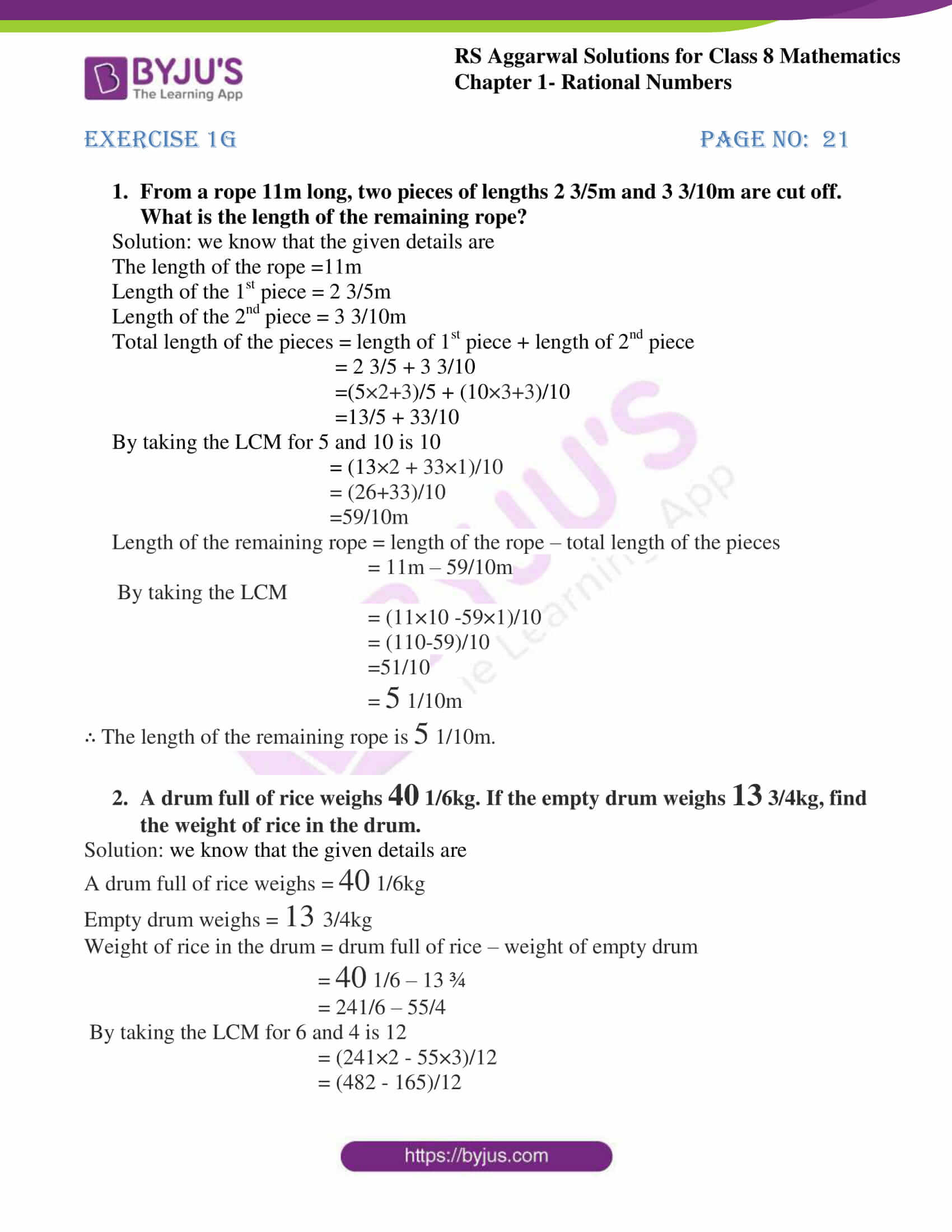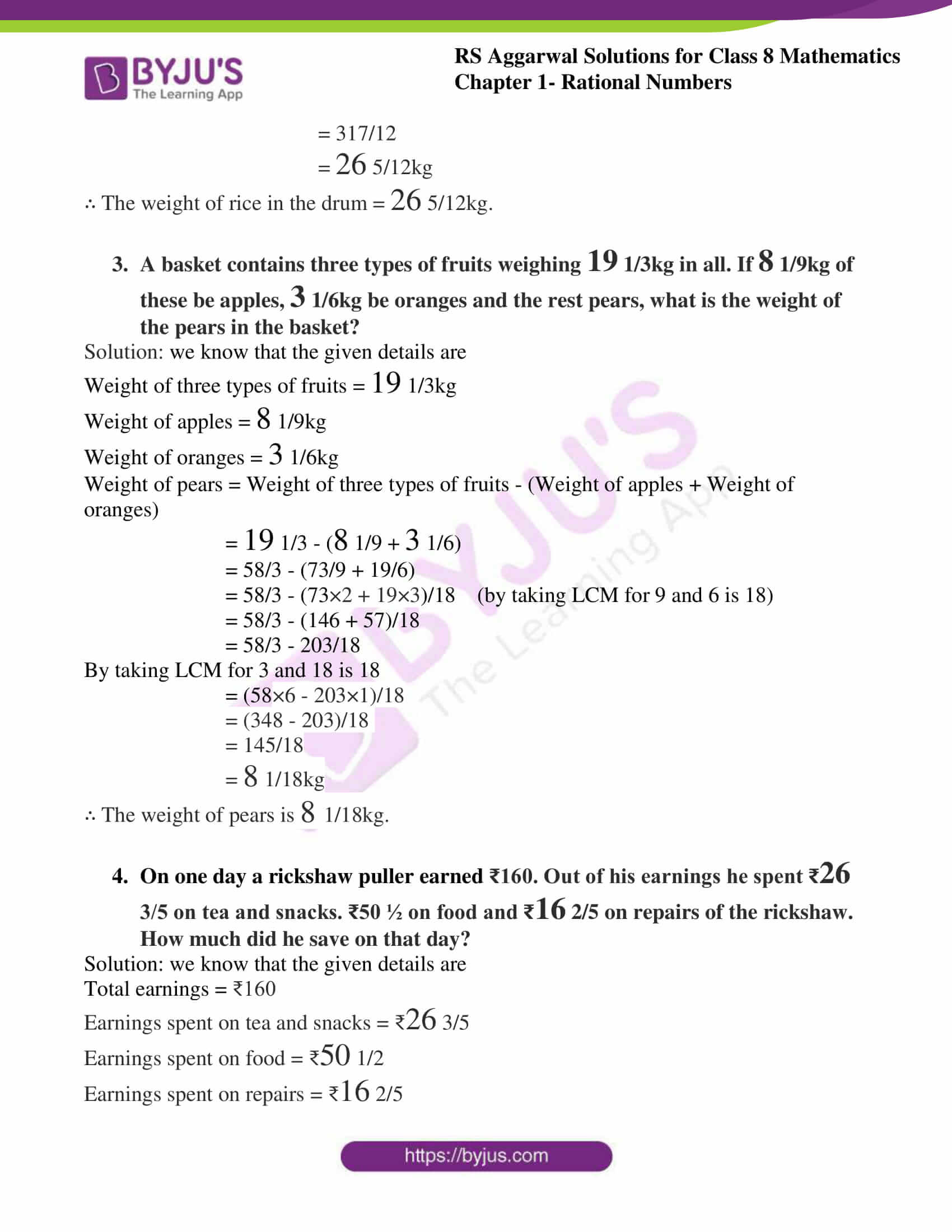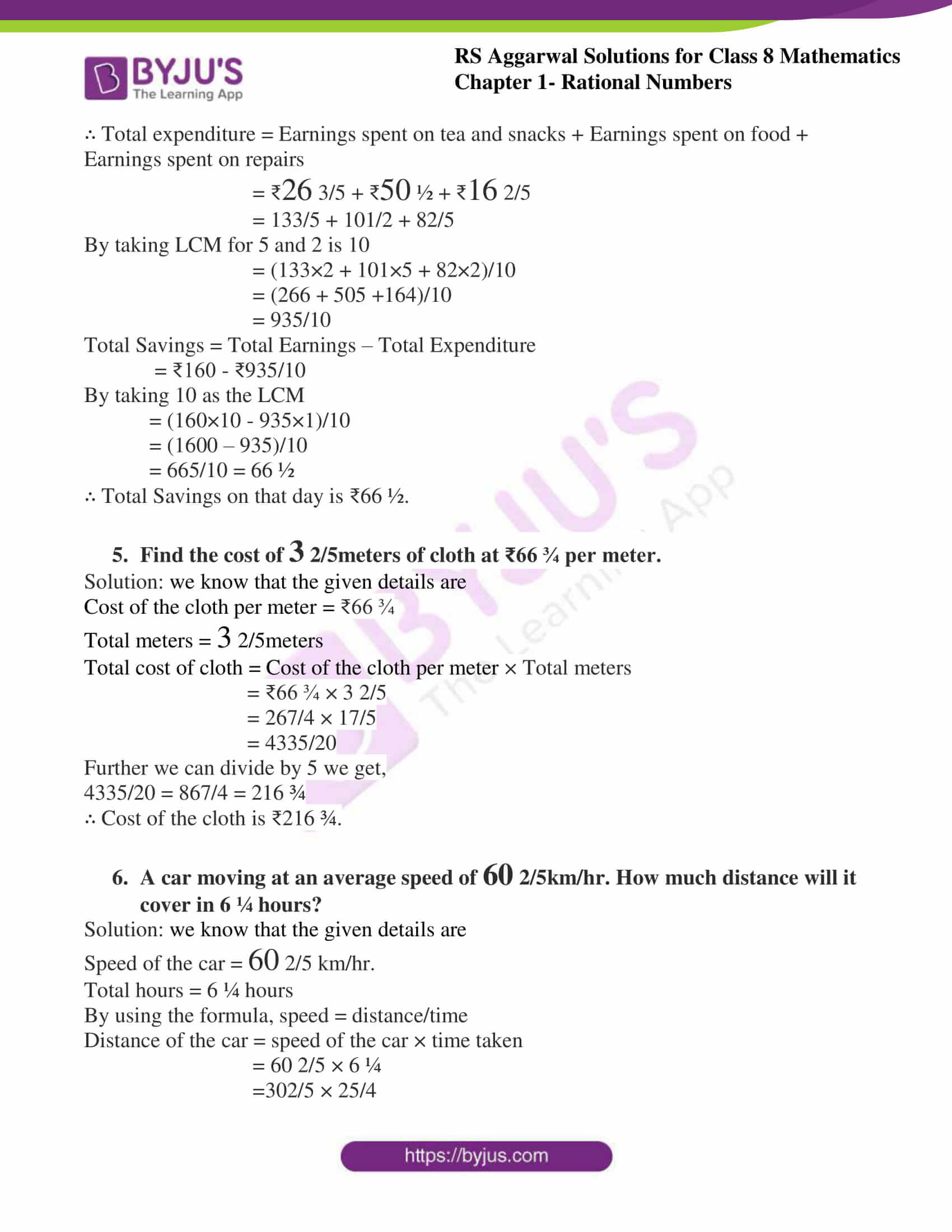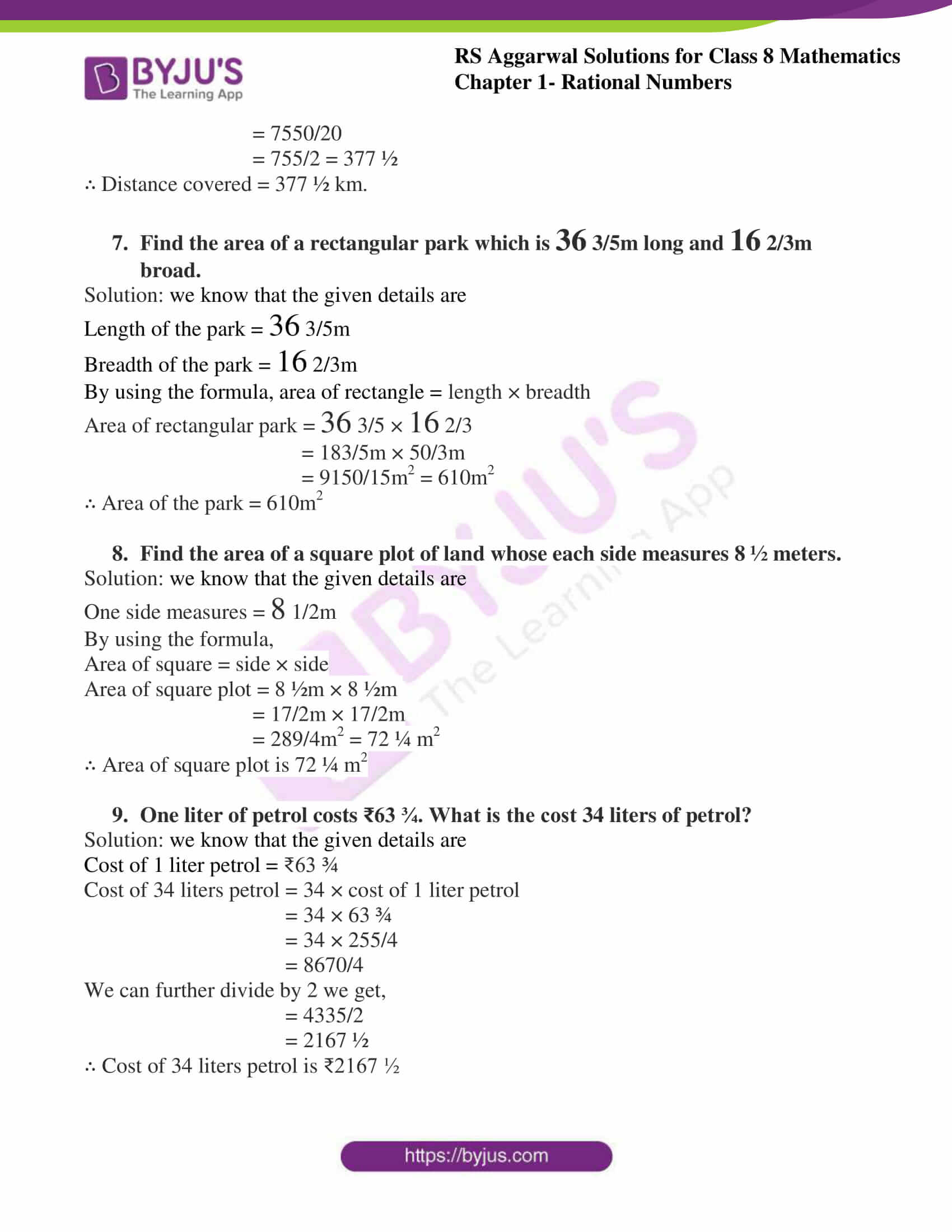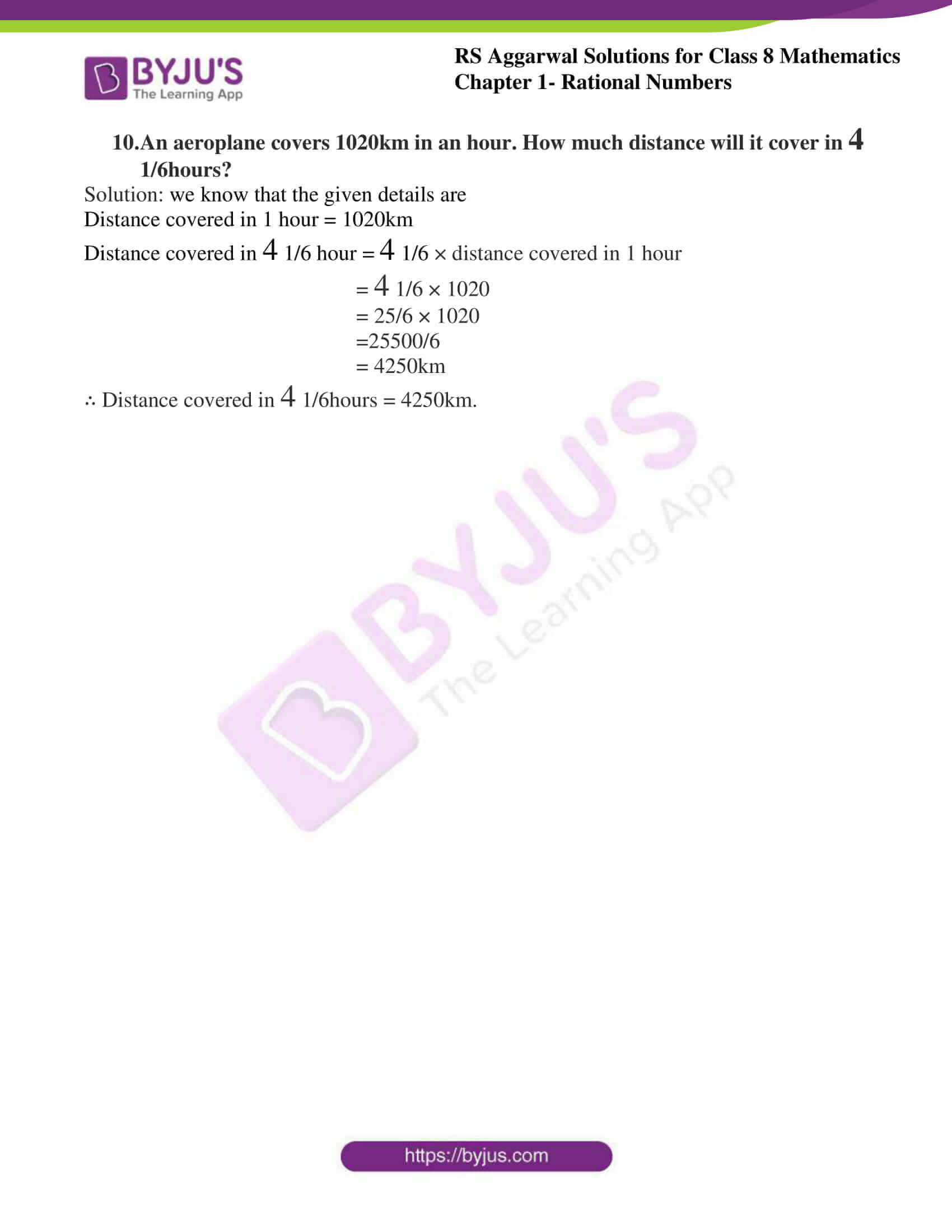### Access answers to RS Aggarwal Solutions for Class 8 Maths Chapter 1- Rational Numbers Exercise 1G

1. From a rope 11m long, two pieces of lengths $2\frac{3}{5}$m and $3\frac{3}{10}$m are cut off. What is the length of the remaining rope?

Solution: we know that the given details are

The length of the rope =11m

Length of the 1st piece = $2\frac{3}{5}$m

Length of the 2nd piece = $3\frac{3}{10}$m

Total length of the pieces = length of 1st piece + length of 2nd piece

= $2\frac{3}{5}$ + $3\frac{3}{10}$

=(5×2+3)/5 + (10×3+3)/10

=13/5 + 33/10

By taking the LCM for 5 and 10 is 10

= (13×2 + 33×1)/10

= (26+33)/10

=59/10m

Length of the remaining rope = length of the rope – total length of the pieces

= 11m – 59/10m

By taking the LCM

= (11×10 -59×1)/10

= (110-59)/10

=51/10

= $5\frac{1}{10}$m

∴ The length of the remaining rope is $5\frac{1}{10}$m.

2. A drum full of rice weighs $40\frac{1}{6}$kg. If the empty drum weighs $13\frac{3}{4}$kg, find the weight of rice in the drum.

Solution: we know that the given details are

A drum full of rice weighs = $40\frac{1}{6}$kg

Empty drum weighs = $13\frac{3}{4}$kg

Weight of rice in the drum = drum full of rice – weight of empty drum

= $40\frac{1}{6}$ – 13 ¾

= 241/6 – 55/4

By taking the LCM for 6 and 4 is 12

= (241×2 – 55×3)/12

= (482 – 165)/12

= 317/12

= $26\frac{5}{12}$kg

∴ The weight of rice in the drum = $26\frac{5}{12}$kg.

3. A basket contains three types of fruits weighing $19\frac{1}{3}$kg in all. If $8\frac{1}{9}$kg of these be apples, $3\frac{1}{6}$kg be oranges and the rest pears, what is the weight of the pears in the basket?

Solution: we know that the given details are

Weight of three types of fruits = $19\frac{1}{3}$kg

Weight of apples = $8\frac{1}{9}$kg

Weight of oranges = $3\frac{1}{6}$kg

Weight of pears = Weight of three types of fruits – (Weight of apples + Weight of oranges)

= $19\frac{1}{3}$ – ($8\frac{1}{9}$ + $3\frac{1}{6}$)

= 58/3 – (73/9 + 19/6)

= 58/3 – (73×2 + 19×3)/18 (by taking LCM for 9 and 6 is 18)

= 58/3 – (146 + 57)/18

= 58/3 – 203/18

By taking LCM for 3 and 18 is 18

= (58×6 – 203×1)/18

= (348 – 203)/18

= 145/18

= $8\frac{1}{18}$kg

∴ The weight of pears is $8\frac{1}{18}$kg.

4. On one day a rickshaw puller earned ₹160. Out of his earnings he spent ₹$26\frac{3}{5}$ on tea and snacks. ₹50 ½ on food and ₹$16\frac{2}{5}$ on repairs of the rickshaw. How much did he save on that day?

Solution: we know that the given details are

Total earnings = ₹160

Earnings spent on tea and snacks = ₹$26\frac{3}{5}$

Earnings spent on food = ₹$50\frac{1}{2}$

Earnings spent on repairs = ₹$16\frac{2}{5}$

∴ Total expenditure = Earnings spent on tea and snacks + Earnings spent on food + Earnings spent on repairs

= ₹$26\frac{3}{5}$+ ₹$50\frac{1}{2}$ + ₹$16\frac{2}{5}$

= 133/5 + 101/2 + 82/5

By taking LCM for 5 and 2 is 10

= (133×2 + 101×5 + 82×2)/10

= (266 + 505 +164)/10

= 935/10

Total Savings = Total Earnings – Total Expenditure

= ₹160 – ₹935/10

By taking 10 as the LCM

= (160×10 – 935×1)/10

= (1600 – 935)/10

= 665/10 = 66 ½

∴ Total Savings on that day is ₹66 ½.

5. Find the cost of $3\frac{2}{5}$ meters of cloth at ₹66 ¾ per meter.

Solution: we know that the given details are

Cost of the cloth per meter = ₹66 ¾

Total meters = $3\frac{2}{5}$meters

Total cost of cloth = Cost of the cloth per meter × Total meters

= ₹66 ¾ × $3\frac{2}{5}$

= 267/4 × 17/5

= 4335/20

Further we can divide by 5 we get,

4335/20 = 867/4 = 216 ¾

∴ Cost of the cloth is ₹216 ¾.

6. A car moving at an average speed of $60\frac{2}{5}$km/hr. How much distance will it cover in 6 ¼ hours?

Solution: we know that the given details are

Speed of the car = $60\frac{2}{5}$ km/hr.

Total hours = 6 ¼ hours

By using the formula, speed = distance/time

Distance of the car = speed of the car × time taken

= $60\frac{2}{5}$× 6 ¼

=302/5 × 25/4

= 7550/20

= 755/2 = 377 ½

∴ Distance covered = 377 ½ km.

7. Find the area of a rectangular park which is $36\frac{3}{5}$m long and $16\frac{2}{3}$m broad.

Solution: we know that the given details are

Length of the park = $36\frac{3}{5}$m

Breadth of the park = $16\frac{2}{3}$m

By using the formula, area of rectangle = length × breadth

Area of rectangular park = $36\frac{3}{5}$ × $16\frac{2}{3}$

= 183/5m × 50/3m

= 9150/15m2 = 610m2

∴ Area of the park = 610m2

8. Find the area of a square plot of land whose each side measures 8 ½ meters.

Solution: we know that the given details are

One side measures = $8\frac{1}{2}$m

By using the formula,

Area of square = side × side

Area of square plot = 8 ½m × 8 ½m

= 17/2m × 17/2m

= 289/4m2 = 72 ¼ m2

∴ Area of square plot is 72 ¼ m2

9. One liter of petrol costs ₹63 ¾. What is the cost 34 liters of petrol?

Solution: we know that the given details are

Cost of 1 liter petrol = ₹63 ¾

Cost of 34 liters petrol = 34 × cost of 1 liter petrol

= 34 × 63 ¾

= 34 × 255/4

= 8670/4

We can further divide by 2 we get,

= 4335/2

= 2167 ½

∴ Cost of 34 liters petrol is ₹2167 ½

10. An aeroplane covers 1020km in an hour. How much distance will it cover in $4\frac{1}{6}$hours?

Solution: we know that the given details are

Distance covered in 1 hour = 1020km

Distance covered in $4\frac{1}{6}$ hour = $4\frac{1}{6}$ × distance covered in 1 hour

= $4\frac{1}{6}$× 1020

= 25/6 × 1020

=25500/6

= 4250km

∴ Distance covered in $4\frac{1}{6}$hours = 4250km.

### Access other Exercises of RS Aggarwal Solutions for Class 8 Maths Chapter 1- Rational Numbers

Exercise 1A Solutions 10 Questions

Exercise 1B Solutions 3 Questions

Exercise 1C Solutions 14 Questions

Exercise 1D Solutions 9 Questions

Exercise 1E Solutions 12 Questions

Exercise 1F Solutions 8 Questions

Exercise 1H Solutions 23 Questions

## RS Aggarwal Solutions for Class 8 Maths Chapter 1- Rational Numbers Exercise 1G

Exercise 1G of RS Aggarwal Class 8 Chapter 1, Rational Numbers contains the advance concepts related to Rational Numbers. This exercise mainly deals with Word problems.

The RS Aggarwal Solutions can help the students practice and learn the fundamentals as it provides all the answers to the questions from the RS Aggarwal textbook. Students should practice RS Aggarwal Solutions without fail to understand how the concepts are solved in order to secure high marks.